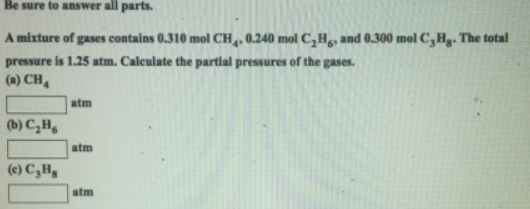# Problem: Be sure to answer all parts.A mixture of gases contains 0.310 mol CH4, 0.240 mol C2H6, and 0.300 mol C3H8. The total pressure is 1.25 atm. Calculate the partial pressures of the gases. (a) CH4 (b) C2H6 (c) C3H8

###### FREE Expert Solution###### Problem Details

Be sure to answer all parts.

A mixture of gases contains 0.310 mol CH4, 0.240 mol C2H6, and 0.300 mol C3H8. The total pressure is 1.25 atm. Calculate the partial pressures of the gases.

(a) CH

(b) C2H6

(c) C3H8